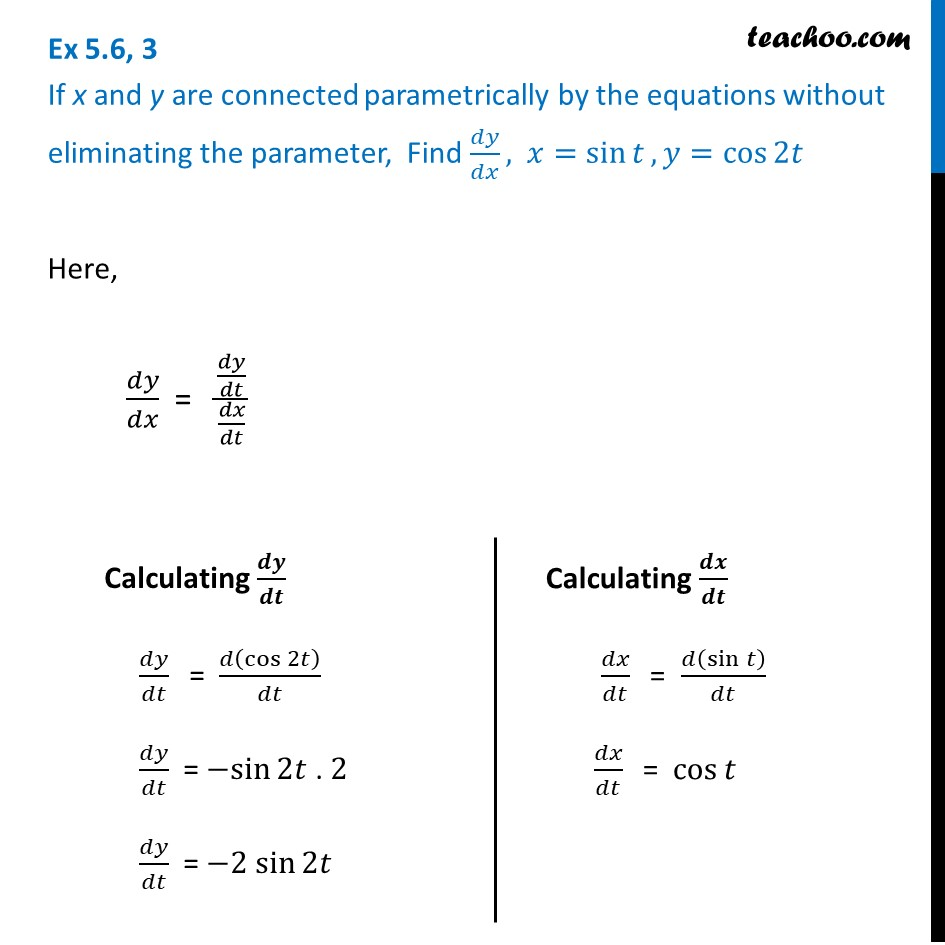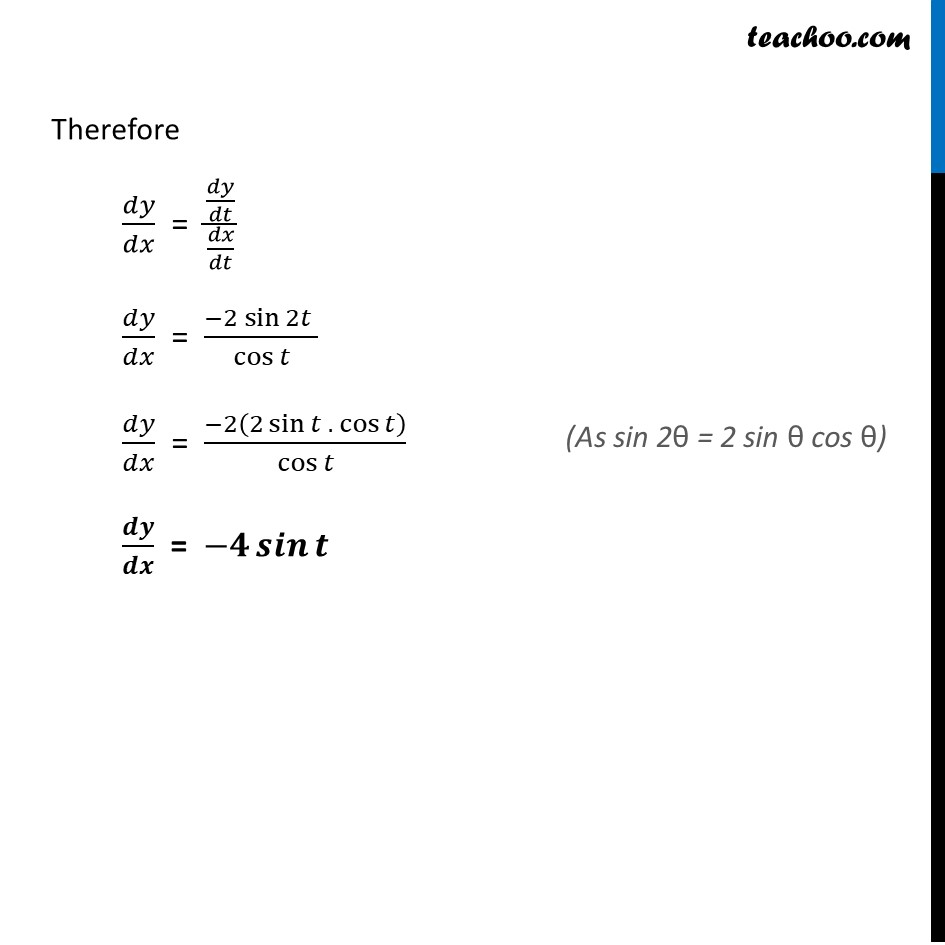Derivatives in parametric form

Chapter 5 Class 12 Continuity and Differentiability
Concept wiseLearn in your speed, with individual attention - Teachoo Maths 1-on-1 Class

### Transcript

Ex 5.6, 3 If x and y are connected parametrically by the equations without eliminating the parameter, Find 𝑑𝑦/𝑑𝑥, 𝑥=sin⁡𝑡, 𝑦=cos⁡2𝑡Here, 𝑑𝑦/𝑑𝑥 = (𝑑𝑦/𝑑𝑡)/(𝑑𝑥/𝑑𝑡) Calculating 𝒅𝒚/𝒅𝒕 𝑑𝑦/𝑑𝑡 " " = 𝑑(cos⁡2𝑡)/𝑑𝑡 𝑑𝑦/𝑑𝑡 = −sin⁡2𝑡 . 2 𝑑𝑦/𝑑𝑡 = −2 sin⁡2𝑡 Calculating 𝒅𝒙/𝒅𝒕 𝑑𝑥/𝑑𝑡 " " = 𝑑(sin⁡𝑡 )/𝑑𝑡 𝑑𝑥/𝑑𝑡 " " = cos⁡𝑡 Therefore 𝑑𝑦/𝑑𝑥 = (𝑑𝑦/𝑑𝑡)/(𝑑𝑥/𝑑𝑡) 𝑑𝑦/𝑑𝑥 = (−2 sin⁡2𝑡" " )/cos⁡𝑡 𝑑𝑦/𝑑𝑥 = (−2(2 sin⁡𝑡 .〖 cos〗⁡𝑡 ))/cos⁡𝑡 𝒅𝒚/𝒅𝒙 = −𝟒 𝒔𝒊𝒏⁡𝒕 (As sin 2θ = 2 sin θ cos θ)